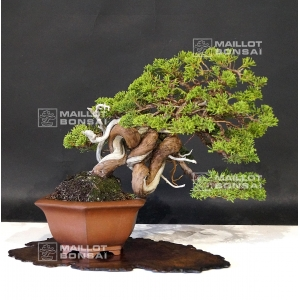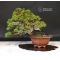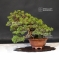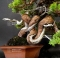##### The Japanese Bonsai specialist
Direct order Contact Help / Services Newsletter# Juniperus chinensis itoigawa ref : 1907194

Juniperus chinensis itoigawaref. : 101861.680,00

Available quantity : 1Order

###### Description

Height of the tree without its pot: 220 mm. Width of the branches: 360 mm. Artisanal quality non-enamelld pot: 190*170*85 mm. Moyogi style. Juniper  with graceful trays, a shari on its twisted trunk and jins. Itoigawa variety with thin green canopy scales, particularly vigorous and perfectly adapted to the bonsaï cultivation. Unlike the classic juniperus chinensis, it only loses little foliage in the beginning of summer. This bonsaï is more than 30/35 years old, that's why its bark is formed.

Trunk diameter of about 30 mm. Nebari of about 70 mm. Not any strong wire wound nor cutting wound. Coming from Master Shiino's nursery Remarkable subject!

Authenthic bonsaï with natural jin and shari, do not mistake with the tanukis.

It was repoted in April 2018 when the trunk was moved to the right, it is necessary to do it again in April 2020.

Admire the knotted trunk, an aspect that makes this bonsaï unique.

The whitening with jin product of the dead trunks, softly tinted with charcoal will give it a  beautiful ash color.

Do you already have this complete book on juniper?

Reshaped in September 2018 with wire by Master Tomoya Nishikawa.

Shelf non-included. Photographed in July 2019.

#with 7.4 #bonsai 6.6 #trunk 4.5 #juniperus 3.9 #chinensis 3.9 #itoigawa 3.8 #this 3.4 #coniferous 3 #master 2.6 #shari 2.5

Formule
(( ROUND((CHAR_LENGTH(b.article_nom)-CHAR_LENGTH(REPLACE(b.article_nom, 'with', '')))/LENGTH('with')) + ROUND((CHAR_LENGTH(b.article_description)-CHAR_LENGTH(REPLACE(b.article_description, 'with', '')))/LENGTH('with')) ) * 7.4) + (( ROUND((CHAR_LENGTH(b.article_nom)-CHAR_LENGTH(REPLACE(b.article_nom, 'bonsai', '')))/LENGTH('bonsai')) + ROUND((CHAR_LENGTH(b.article_description)-CHAR_LENGTH(REPLACE(b.article_description, 'bonsai', '')))/LENGTH('bonsai')) ) * 4.6) + (( ROUND((CHAR_LENGTH(b.article_nom)-CHAR_LENGTH(REPLACE(b.article_nom, 'trunk', '')))/LENGTH('trunk')) + ROUND((CHAR_LENGTH(b.article_description)-CHAR_LENGTH(REPLACE(b.article_description, 'trunk', '')))/LENGTH('trunk')) ) * 4.5) + (( ROUND((CHAR_LENGTH(b.article_nom)-CHAR_LENGTH(REPLACE(b.article_nom, 'juniperus', '')))/LENGTH('juniperus')) + ROUND((CHAR_LENGTH(b.article_description)-CHAR_LENGTH(REPLACE(b.article_description, 'juniperus', '')))/LENGTH('juniperus')) ) * 3.9) + (( ROUND((CHAR_LENGTH(b.article_nom)-CHAR_LENGTH(REPLACE(b.article_nom, 'chinensis', '')))/LENGTH('chinensis')) + ROUND((CHAR_LENGTH(b.article_description)-CHAR_LENGTH(REPLACE(b.article_description, 'chinensis', '')))/LENGTH('chinensis')) ) * 3.9) + (( ROUND((CHAR_LENGTH(b.article_nom)-CHAR_LENGTH(REPLACE(b.article_nom, 'itoigawa', '')))/LENGTH('itoigawa')) + ROUND((CHAR_LENGTH(b.article_description)-CHAR_LENGTH(REPLACE(b.article_description, 'itoigawa', '')))/LENGTH('itoigawa')) ) * 3.8) + (( ROUND((CHAR_LENGTH(b.article_nom)-CHAR_LENGTH(REPLACE(b.article_nom, 'this', '')))/LENGTH('this')) + ROUND((CHAR_LENGTH(b.article_description)-CHAR_LENGTH(REPLACE(b.article_description, 'this', '')))/LENGTH('this')) ) * 3.4) + (( ROUND((CHAR_LENGTH(b.article_nom)-CHAR_LENGTH(REPLACE(b.article_nom, 'master', '')))/LENGTH('master')) + ROUND((CHAR_LENGTH(b.article_description)-CHAR_LENGTH(REPLACE(b.article_description, 'master', '')))/LENGTH('master')) ) * 2.6) + (( ROUND((CHAR_LENGTH(b.article_nom)-CHAR_LENGTH(REPLACE(b.article_nom, 'shari', '')))/LENGTH('shari')) + ROUND((CHAR_LENGTH(b.article_description)-CHAR_LENGTH(REPLACE(b.article_description, 'shari', '')))/LENGTH('shari')) ) * 2.5) + (( ROUND((CHAR_LENGTH(b.article_nom)-CHAR_LENGTH(REPLACE(b.article_nom, 'about', '')))/LENGTH('about')) + ROUND((CHAR_LENGTH(b.article_description)-CHAR_LENGTH(REPLACE(b.article_description, 'about', '')))/LENGTH('about')) ) * 2.5)

## Secure payment## Delivery

Our logistic partners :